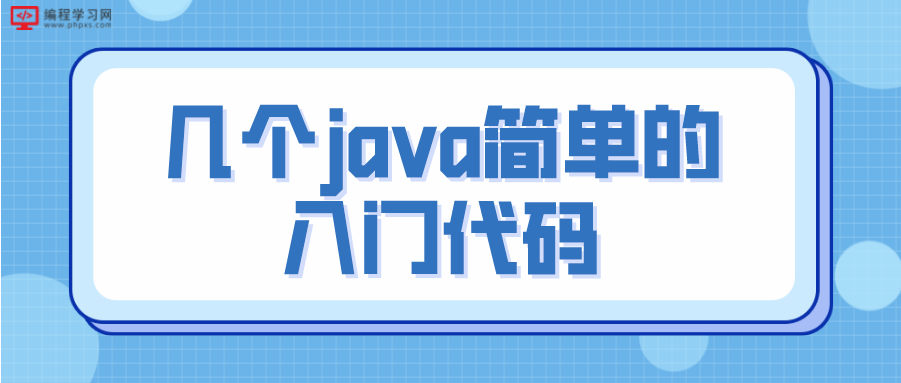2023
03-01

# 几个java简单的入门代码（Java简单入门代码整理）package com.hpe.java;//这是一个问好程序public class Hello {
//一个类只能有一个main方法
public static void main(String arg[]) {
System.out.print("helloworld!");//输出不换行
System.out.println("heng");//输出换行
}}

helloworld!heng

package com.hpe.java;
public class Demo1 {
/*
* 什么是常量？就是程序运行期间，固定的值是不能改变的
*/
public static void main(String arg[]) {
//常量有多少种？
//1.整数
System.out.println(100);
//2.小数
System.out.println(250.250);
//3.字符串
System.out.println("字符");
//4.字符
System.out.println('我');//字符只能写一个，并且不能为空

System.out.println("=============");
//5.布尔：只能有两个值，要么是true要么就是false
System.out.println(false);
}}

100
250.25

=============
false

package com.hpe.java;
public class Demo2 {
/*
* 基本数据类型
* 整数类型：byte short int(常用) long
* 浮点类型：float double(默认)
* 字符类型：char
* 布尔类型：boolean
* 引用类型
* 数据类型除了8种基本数据类型，剩下的全是引用类型
*/
public static void main(String arg[]) {
//定义变量有两种方式
/*
* 第一种方式：
* 数据类型  变量名；
* 变量名=数据值；
*/
byte num1;//创建了一个byte类型的变量，名称叫num1
num1=100;//为变量赋值，把右边的常量100赋值给了左侧的变量
System.out.println("num1="+num1);
//改变变量num1的值
num1=111;
//num1=128; //byte的存储范围在-128~127，右侧进行赋值的数据不能超过左侧变量数据类型范围
System.out.println("num1="+num1);
/*
* 创建变量的第二种方式
* 数据类型 变量名=数值；创建变量的同时给变量赋值
*/
byte num2=109;
System.out.println("num2="+num2);

//long类型
long num3=3456;//如果不加l或L默认是int类型
System.out.println("num3="+num3);

//浮点数
double num4=123;
System.out.println("num4="+num4);//123.0
float num5=1234f;//后面加上F或者f代表是float
System.out.println("num5="+num5);//1234.0

//字符
char c='a';//只能是一个字符
System.out.println("c="+c);
//布尔类型：只能是true或者false，常用于逻辑判断
boolean b=true;
//基本数据类型转换
/*
* 小范围到大范围，自动类型转换
*/
short s=123;
int num=s;
System.out.println("num="+num);
/*
* 强制类型转换：大范围换小范围
*/
int i=345;
short s1=(short)i;
System.out.println(s1);
}}

num1=100
num1=111
num2=109
num3=3456
num4=123.0
num5=1234.0
c=a
num=123
345